# Physics: Post your doubts here!

#### Omkar Kerkar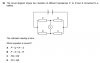From O/N 2017 paper 11
The circuit diagram shows four resistors of different resistances P,Q,R and S connected to a battery. (SEE IMAGE) The voltmeter reading is zero. Which equation is correct?
A) P - Q = R - S
B) P - S = Q - R
C) PQ = RS
D) PS = QR

THANKS FOR THE HELP IN ADVANCELast edited by a moderator:

#### PlanetMaster

View attachment 64586

From O/N 2017 paper 11
The circuit diagram shows four resistors of different resistances P,Q,R and S connected to a battery. (SEE IMAGE) The voltmeter reading is zero. Which equation is correct?
A) P - Q = R - S
B) P - S = Q - R
C) PQ = RS
D) PS = QR

THANKS FOR THE HELP IN ADVANCE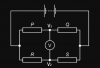Since V=0 therefore V1=V2.

Since PQ resistor system is essentially a potential divider, we have for V1: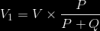and similarly for V2:Since V=0 therefore V1=V2. Substituting V1 and V2 gives us: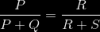And equating these gives us: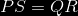Hope this helps.#### Omkar Kerkar

View attachment 64587

Since V=0 therefore V1=V2.

Since PQ resistor system is essentially a potential divider, we have for V1:

View attachment 64588

and similarly for V2:

View attachment 64591

Since V=0 therefore V1=V2. Substituting V1 and V2 gives us:

View attachment 64592

And equating these gives us:

View attachment 64593

Hope this helps.RIGHT! MAkes sense, tysm#### hellodjfos;s'ff

You have either posted the wrong link or posted in the wrong thread.

Edit: Regardless of that, I assume you are asking about s16 qp41 q1b(ii) - physics.
If that's the case, in part b(ii) we are trying to find the minimum speed required for the rock to reach star B, so the rock must reach the point where the gravitational attraction is zero. This is the point where the forces of attraction on the rock by stars A and B are equal and after this point, the rock will get pulled by star B. If you observe the graph, you will notice that the point where the potential begins to change direction is near x=0.52 or 0.54. At this point the potential is -4.4, so if you find the change in GPE between potential=-10 and potential=-4.4, you will get the change in GPE needed to reach star B. And since change in GPE=change in KE, you can now find the velocity.

Last edited:

#### MShaheerUddin

You have either posted the wrong link or posted in the wrong thread.

Edit: Regardless of that, I assume you are asking about s16 qp41 q1b(ii) - physics.
If that's the case, in part b(ii) we are trying to find the minimum speed required for the rock to reach star B, so the rock must reach the point where the gravitational attraction is zero. This is the point where the forces of attraction on the rock by stars A and B are equal and after this point, the rock will get pulled by star B. If you observe the graph, you will notice that the point where the potential begins to change direction is near x=0.52 or 0.54. At this point the potential is -4.4, so if you find the change in GPE between potential=-10 and potential=-4.4, you will get the change in GPE needed to reach star B. And since change in GPE=change in KE, you can now find the velocity.
Thanks bro but still i am not clear that why for minimum velocity it has to reach to point where gravitational attraction is zero

#### PlanetMaster

Thanks bro but still i am not clear that why for minimum velocity it has to reach to point where gravitational attraction is zero
Please provide a link to the question. The link you provided earlier seems to be for a different subject.

#### hellodjfos;s'ff

Thanks bro but still i am not clear that why for minimum velocity it has to reach to point where gravitational attraction is zero
Star A Rock Zero GPE point Star B
I'll assume that above are the positions of the objects. So Between Star A and zero GPE point, the star A is trying to pull the rock towards itself so the rock must have enough KE to reach zero GPE point for it to leave star A's gravitational attraction. After zero GPE point, the star B will automatically try to pull the rock towards itself so the rock can even be stationary after zero GPE point and it will reach star B. So we need to find the change in GPE from Star A to zero GPE point because that is the change in KE that will take place for the rock to come within reach of star B's gravitational pull.

•MShaheerUddin

#### MShaheerUddin

Star A Rock Zero GPE point Star B
I'll assume that above are the positions of the objects. So Between Star A and zero GPE point, the star A is trying to pull the rock towards itself so the rock must have enough KE to reach zero GPE point for it to leave star A's gravitational attraction. After zero GPE point, the star B will automatically try to pull the rock towards itself so the rock can even be stationary after zero GPE point and it will reach star B. So we need to find the change in GPE from Star A to zero GPE point because that is the change in KE that will take place for the rock to come within reach of star B's gravitational pull.
Thanks a lot#### hellodjfos;s'ff

View attachment 64643
B(i) only
So P has to be that curve since P is the first nuclide that begins decaying so it must have existing amount hence it has a non-zero y intercept. D has to be the curve with an increase and decrease because at first when P decays, the rate of formation of D is high so there are lots of D nuclide being made but after a while the D nuclide also begins decaying into S nuclide so it has to decrease in amount later. S is always increasing because when P decays, it forms D and when D decays it forms S so all the previous nuclide are eventually decaying to form S hence its amount will increase always.

#### Attachments

•MShaheerUddin

#### hellodjfos;s'ff

Also help with this part cView attachment 64645
This would be my official answer to c: Decay constant is probability of decay per unit time. Since E has a smaller decay constant, it is less likely to decay into F and since F has a larger decay constant it is more likely to decay into G. Hence, the amount of F is always small as the rate of decay of F is greater than rate of formation of F. Alternatively, greater decay constant means lower half life(inverse relation), hence E has a greater half life and F has a lower half life, thus E decays less often to form F while F decays quickly form G. Therefore, F is always small as again rate of decay of F is greater than its rate of formation.

•MShaheerUddin

#### MShaheerUddin

This would be my official answer to c: Decay constant is probability of decay per unit time. Since E has a smaller decay constant, it is less likely to decay into F and since F has a larger decay constant it is more likely to decay into G. Hence, the amount of F is always small as the rate of decay of F is greater than rate of formation of F. Alternatively, greater decay constant means lower half life(inverse relation), hence E has a greater half life and F has a lower half life, thus E decays less often to form F while F decays quickly form G. Therefore, F is always small as again rate of decay of F is greater than its rate of formation.
Thank a lot .. you are genius!

•hellodjfos;s'ff

#### hellodjfos;s'ff

View attachment 64652 can you help me with last part iii
The question asks for the time between the minimum and maximum frequency which would be 0.5period of the information signal.
The information signal has a frequency of 10kHz so this is the frequency at which the wave operates. Use f=1/T to find the period and half that to get the time.

•MShaheerUddin

#### lolo lolo

I need physics paper4 march 2019 paper for IGCSE 0625